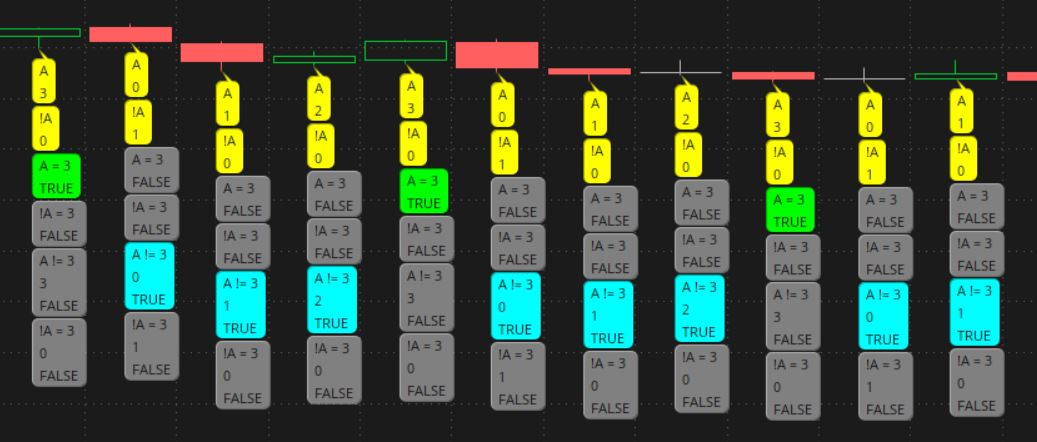# Comparison Operators

#### Kden

##### New member
What is different between:
if A != 3; and if !A == 3;

Thank you;
Kden

Solution
What is different between:
if A != 3; and if !A == 3;

Thank you;
Kden

this is a test study that compares variable A and !A in some formulas.
variable A counts 0 , 1 , 2 , 3
draw 6 bubbles, with values of A and various conditions

A != 3
when is A not equal to 3? when it is 0,1,2

!A == 3
when is not A equal to 3? never. !0 = 1 , !1 = 0 , !2 = 0 , !3 = 0

Ruby:
``````# test_not1

# What is different between:
# if A != 3; and if !A == 3;

# test_not1
def bn = BarNumber();
#  a is a count number  0,1,2,3
def a = (bn % 4);
def not_equal = ( a != 3);
def not_a = ( !A == 3);

#-----------------------------

# bubble legend
What is different between:
if A != 3; and if !A == 3;

Thank you;
Kden

this is a test study that compares variable A and !A in some formulas.
variable A counts 0 , 1 , 2 , 3
draw 6 bubbles, with values of A and various conditions

A != 3
when is A not equal to 3? when it is 0,1,2

!A == 3
when is not A equal to 3? never. !0 = 1 , !1 = 0 , !2 = 0 , !3 = 0

Ruby:
``````# test_not1

# What is different between:
# if A != 3; and if !A == 3;

# test_not1
def bn = BarNumber();
#  a is a count number  0,1,2,3
def a = (bn % 4);
def not_equal = ( a != 3);
def not_a = ( !A == 3);

#-----------------------------

# bubble legend

# -----------------------------

"A" + "\n" +
a
, color.yellow, no);

"!A" + "\n" +
!a
, color.yellow, no);

"A = 3" + "\n" +
(if a == 3 then "TRUE" else "FALSE")
, (if a == 3 then color.green else color.gray), no);

"!A = 3" + "\n" +
(if !a == 3 then "TRUE" else "FALSE")
, (if !a == 3 then color.green else color.gray), no);

"A != 3" + "\n" +
a + "\n" +
(if (a != 3) then "TRUE" else "FALSE")
, (if (a != 3) then color.cyan else color.gray), no);

"!A = 3" + "\n" +
!a + "\n" +
(if (!A == 3) then "TRUE" else "FALSE")
, (if (!A == 3) then color.blue else color.gray), no);

# ----------------------------

bn + " bar#\n" +
a + " A\n" +
!a + " !A\n" +
not_equal + " A!=3\n" +
not_a + " !A=3"
, color.yellow, no);
#``````

create a variable A, that counts 0 , 1 , 2 , 3
draw 6 bubbles, with values of A and various conditionshal_not

Last edited:
•MerryDay

### Not the exact question you're looking for?

87k+ Posts
168 Online## The Market Trading Game Changer

Join 2,500+ subscribers inside the useThinkScript VIP Membership Club
• Exclusive indicators
• Proven strategies & setups
• Private Discord community
• Exclusive members-only content
• 1 full year of unlimited support

What is useThinkScript?

useThinkScript is the #1 community of stock market investors using indicators and other tools to power their trading strategies. Traders of all skill levels use our forums to learn about scripting and indicators, help each other, and discover new ways to gain an edge in the markets.

How do I get started?

We get it. Our forum can be intimidating, if not overwhelming. With thousands of topics, tens of thousands of posts, our community has created an incredibly deep knowledge base for stock traders. No one can ever exhaust every resource provided on our site.

If you are new, or just looking for guidance, here are some helpful links to get you started.

What are the benefits of VIP Membership?